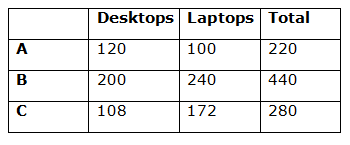# IBPS SO Prelims Quantitative Aptitude Questions 2019 (Day-6)

Dear Aspirants, Our IBPS Guide team is providing new series of Quantitative Aptitude Questions for IBPS SO Prelims 2019 so the aspirants can practice it on a daily basis. These questions are framed by our skilled experts after understanding your needs thoroughly. Aspirants can practice these new series questions daily to familiarize with the exact exam pattern and make your preparation effective.

Ensure Your Ability Before of Exam

Take IBPS SO Prelims Free Mock test

[WpProQuiz 7541]

Caselet

Directions (1 – 5): Read the following information carefully and answer the given question.

There are three companies A, B and C sold laptops and desktops in January 2019.

Number of laptops sold in company C is 28 less than the number of desktops sold in company B. Number of laptops sold in company B is 240, which is 20% more than the number of desktops sold in the same company.

Total number of items sold in company A is 50% of the total number of items sold in company B. Number of desktops sold in company A is half of the number of laptops sold in company B.

The ratio of the number of laptops sold in company A to that of the number of desktops sold in company C is 25: 27.

1) Number of laptops sold in company A is what percentage more/less than number of desktops sold in company B?

a) 50% more

b) 30% less

c) 40% more

d) 50% less

e) None of these

2) What is the ratio of the total number of desktops sold in all the companies together to that of the total number of items sold in company C?

a) 113: 79

b) 119: 73

c) 117: 70

d) 103: 79

e) None of these

3) What is the difference between the total number of items sold in company A to that of C?

a) 40

b) 60

c) 30

d) 50

e) 72

4) If company D sold number of desktops is 20% more than that of company A and the ratio of the number of desktops sold in company D to that of laptops is 2: 3. What is the total number of items sold in company D?

a) 360

b) 240

c) 300

d) 320

e) 400

5) If 20% of the laptops sold in company B is defective, find the number of non – defective laptops sold in company B?

a) 216

b) 180

c) 196

d) 172

e) 192

Wrong numbers

Directions (6 – 10): Find the wrong number in the following number series.

6) 2, 123, 269, 436, 632

a) 269

b) 123

c) 632

d) 2

e) 436

7) 3, 33, 75, 132, 203

a) 3

b) 132

c) 33

d) 75

e) 203

8) 5832, 6854, 7993, 9252, 10637

a) 6854

b) 9252

c) 5832

d) 7993

e) 10637

9)  7993, 8202, 8057, 8226, 8105

a) 8057

b) 7993

c) 8226

d) 8202

e) 8105

10) 20, 42, 130, 520, 2605

a) 42

b) 520

c) 2605

d) 20

e) 130

Directions (1 – 5):

Number of laptops sold in company B = 240

Number of desktops sold in company B = 240 * (100/120) = 200

Number of laptops sold in company C = 200 – 28 = 172

Total number of items sold in company B = 240 + 200 = 440

Total number of items sold in company A = 440 * 50/100 = 220

Number of desktops sold in company A = ½ * 240 = 120

Number of laptops sold in company A = 220 – 120 = 100

Number of desktops sold in company C = 100 * (27/25) = 108Required percentage = (200 – 100)/200 * 100

= 100/200 * 100 = 50% less

Required ratio = (120 + 240 + 108): 280

= 468: 280

= 117: 70

Required ratio = 280 – 220 = 60

Number of desktops sold in company D = 120 * (120/100) = 144

Number of laptops sold in company D = 144 * (3/2) = 216

Required total = 144 + 216 = 360

Required number of laptops = 240 * 80/100 = 192

Directions (6-10) :

2 + 112 = 123

123 + 122 = 267 (not 269)

267 + 132 = 436

436 + 142 = 632

3 + (5 * 6) = 33

33 + (6 * 7) = 75

75 + (7 * 8) = 131 (not 132)

131 + (8 * 9) = 203

183 – 3 = 5829 (not 5832)

193 – 5 = 6854

203 – 7 = 7993

213 – 9 = 9252

223 – 11 = 10637

7993 + 172 = 8282 (not 8202)

8282 – 152 = 8057

8057 + 132 = 8226

8226 – 112 = 8105

(20 * 2) + 2 = 42

(42 * 3) + 3 = 129 (not 130)

(129 * 4) + 4 = 520

(520 * 5) + 5 = 2605